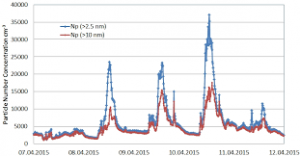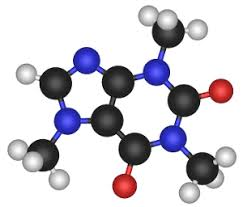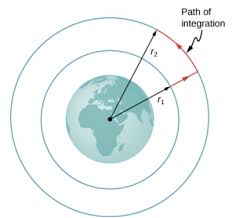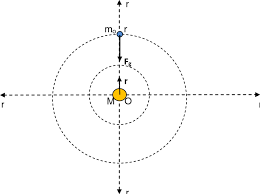## How to Calculate and Solve for the Mass, Volume and Mass Concentration in Chemistry | The Calculator EncyclopediaThe image above represents the mass concentration.

To compute the mass concentration, two essential parameters are needed and these parameters are mass (m) and volume (V).

The formula for calculating mass concentration:

ρ = mV

Where;
ρ = Mass concentration
m = Mass
V = Volume

Let’s solve an example;
Find the mass concentration when the mass is 8 kg with a volume of 24 dm³.

This implies that;
m = Mass = 8
V = Volume = 24

ρ = mV
ρ = 824
ρ = 0.33

Therefore, the mass concentration is 0.33 Kg/dm³.

Calculating the Mass when the Mass Concentration and the Volume is Given.

m = Vρ

Where;
m = Mass
ρ = Mass concentration
V = Volume

Let’s solve an example;
Find the mass when the mass concentration is 12 kg/dm³ with a volume of 7 dm³.

This implies that;
ρ = Mass concentration = 12
V = Volume = 7

m = Vρ
m = 12 x 7
m = 84

Therefore, the mass concentration is 84 kg.

## How to Calculate and solve for the Mass, Number of Moles and Molar Mass in Chemistry | The Calculator EncyclopediaThe image above represents the molar mass of a substance.

To compute for the molar mass, two essential parameters are needed and these parameters are mass (m) and number of moles (n).

The formula for calculating the molar mass:

M = mn

Where;
M = Molar mass
m = Mass
n = Number of moles

Let’s solve an example;
Find the molar mass of a substance with a mass of 14 kg and number of moles of 28 mol.

This implies that;
m = Mass = 14
n = Number of moles = 28

M = mn
M = 14 ⁄ 28
M = 0.5

Therefore, the molar mass is 0.5 Kg/mol.

Calculating the Mass of a Substance when the Molar Mass and the Number of Moles is Given.

m = nM

Where;
m = Mass
n = Number of moles
M = Molar Mass

Let’s solve an example;
Find the mass of a substance with a molar mass of 30 Kg/mol and number of moles of 15 mol.

This implies that;
n = Number of moles = 15
M = Molar Mass = 30

m = nM
m = 15 x 30
m =  450

Therefore, the mass of a substance is 450 kg.

## How to Calculate and Solve for Gravitational Potential | The Calculator EncyclopediaThe image above represents the Gravitational potential.

To compute the gravitational potential of a field, two essential parameters are needed which are mass (m) and radius (r).

The formula for calculating the gravitational potential;

V = (Gm) / r

Where;
V = Gravitational potential
m = Mass

Let’s solve an example;
Find the gravitational potential of a field when the mass is 14 cm with a radius of 9 cm.

This implies that;
m = Mass = 14 cm
r = Radius = 9 cm

V = (Gm) / r
V = (6.67 x 10-11 x 14) / 9
V = 1.0375e-10 / 9
V = 1.0375e-10

Therefore, the gravitational potential is 1.0375e-10 Volts (V).

## How to Calculate and Solve for Gravitational Force | The Calculator EncyclopediaThe image above represents the gravitational force.

To compute the gravitational force of a field, three parameters are needed and this parameters are mass (m1), mass (m2) and radius between the masses (R).

The formula for calculating the gravitational force:

F = Gm1m2 /

Where;
F = Gravitational force
m1 = Mass 1
m2 = Mass 2
r = Radius between the masses

Let’s solve an example;
Find the gravitational force of a field when the mass 1 is 8 cm, mass 2 is 10 cm and the radius between masses is 14 cm.

This implies that;
m1 = Mass 1 = 8 cm
m2 = Mass 2 = 10 cm
r = Radius between the masses = 14 cm

F = Gm1m2 /
F = (6.67 x 10-11 x 8 x 10) / 196
F = 5.336e-9 / 196
F = 2.722e-11

Therefore, the gravitational force is 2.722e-11 Newton (N).

## The Calculator Encyclopedia Calculates and Solves the Wavelength for a Wave – Particle Behaviour

According to Wikipedia,

Wave–particle duality is the concept in quantum mechanics that every particle or quantum entity may be partly described in terms not only of particles, but also of waves.

In physics, the wavelength is the spatial period of a periodic wave—the distance over which the wave’s shape repeats.

Nickzom Calculator requires two parameters to compute the wavelength of a wave or particle. These parameters are:

• Mass
• Velocity

The formula for computing the wavelength is:

λ = h / mv

Where:
λ = Wavelength
h = Planck’s Constant (6.63 x 10-34 js)
m = Mass
v = Velocity

Let’s solve an example, find the wavelength of a wave – particle with a mass of 300 Kg and a velocity of 3 x 1010 m/s.

From the example, we can see that:
m = 300
v = 3 x 1010

λ = 6.63 x 10-34 / 300 (3 x 1010)
λ = 6.63 x 10-34 / 9 x 1012
λ = 7.37 x 10-47

Therefore, the wavelength of the wave – particle (λ) is 7.37 x 10-47.